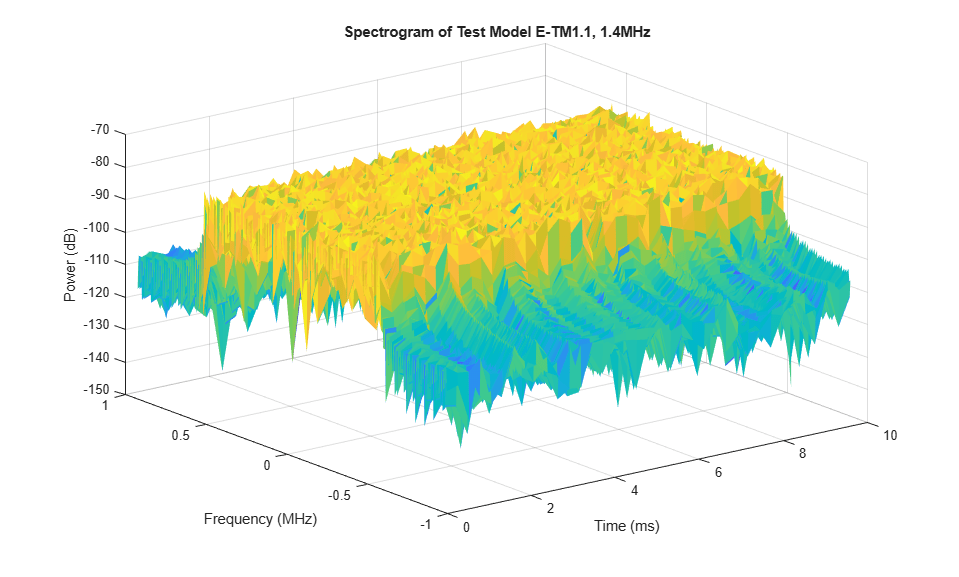# LTE Downlink Test Model (E-TM) Waveform Generation

This example shows how to generate a test model using LTE Toolbox™.

### Overview

The LTE specifications define conformance test models for transmitter tests. These include transmit signal quality, output power dynamics, Error Vector Magnitude (EVM) for various modulation schemes, Base Station (BS) output power, Reference Symbol (RS) absolute accuracy, etc. This example demonstrates how these different test model waveforms can be generated using LTE Toolbox functions.

The following general parameters are used by all E-UTRA test models as defined in TS 36.141, Section 6.1.2 [ 1 ] :

• Single antenna port, 1 code word, 1 layer without any precoding

• Duration is 10 subframes (10 ms)

• Normal cyclic prefix

• Virtual resource blocks of localized type

• User Equipment (UE)-specific reference signals are not used

The following physical channels and signals will be generated:

• Reference Signals (CellRS)

• Primary Synchronization Signal (PSS)

• Secondary Synchronization Signal (SSS)

• Physical Control Format Indicator Channel (PCFICH)

• Physical Hybrid-ARQ Indicator Channel (PHICH)

• Physical Downlink Control Channel (PDCCH)

• Physical Downlink Shared Channel (PDSCH)

Test models are selected according to required test cases. In our example, the considered test model, E-TM1.1, should be used for tests on:

• BS output power

• Unwanted emissions - Occupied bandwidth, Adjacent Channel Leakage power Ratio (ACLR), operating band unwanted emissions, transmitter spurious emissions

• Transmitter intermodulation

• Reference signals absolute accuracy

### Test Model Selection

A number of test models are defined in TS 36.141 Section 6.1 [ 1 ]. This example will generate test model 1.1 as shown below.

```tm = '1.1'; % Test model number ```

Allowed test model values in the toolbox are ('1.1', '1.2', '2', '2a', '3.1', '3.1a', '3.2', '3.3').

### Bandwidth Selection

The test model generation function in the toolbox requires the bandwidth to be specified as shown below:

```bw = '1.4MHz'; % Bandwidth ```

### Test Model Generation

The channel model number and the bandwidth determine the physical channel and signal parameters as specified in TS 36.141. The generated waveform `timeDomainSig` is a time domain signal after performing OFDM modulation, cyclic prefix insertion and windowing. `txGrid` is a 2-dimensional array representing the resource grid spanning 10 subframes.

```[timeDomainSig, txGrid, txInfo] = lteTestModelTool(tm,bw); ```

### Plot Transmitted Resource Grid

Plot the resource grid `txGrid`, with a legend describing which resource elements are allocated to which physical channels and signals.

```hPlotDLResourceGrid(txInfo,txGrid); ```### Plot Spectrogram

Plot a spectrogram of the time domain signal.

```% Compute spectrogram [y,f,t,p] = spectrogram(timeDomainSig, 512, 0, 512, txInfo.SamplingRate); % Re-arrange frequency axis and spectrogram to put zero frequency in the % middle of the axis i.e. represent as a complex baseband waveform f = (f-txInfo.SamplingRate/2)/1e6; p = fftshift(10*log10(abs(p))); % Plot spectrogram figure; surf(t*1000,f,p,'EdgeColor','none'); xlabel('Time (ms)'); ylabel('Frequency (MHz)'); zlabel('Power (dB)'); title(sprintf('Spectrogram of Test Model E-TM%s, %s',tm, bw)); ```### Further Exploration

For over-the-air transmission and analysis of the test model waveform, refer to the following example: Waveform Generation and Transmission Using LTE Toolbox with Test and Measurement Equipment.

### Appendix

This example uses the following helper function:

### Selected Bibliography

1. 3GPP TS 36.141 "Base Station (BS) conformance testing"